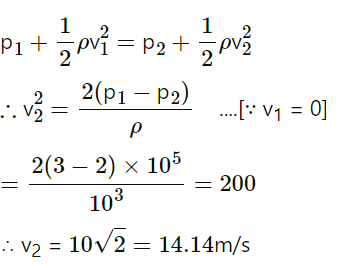# The pressure of water inside the closed pipe is 3 x105 N/m2. This pressure reduces to 2x105 N/m2 on opening the value of the pipe.

more_vert

The pressure of water inside the closed pipe is 3 x105 N/m2. This pressure reduces to 2 x105 N/m2 on opening the value of the pipe. Calculate the speed of water flowing through the pipe. (Density of water = 1000 kg/m3)

more_vert

verified

Explaination::

Given::  p1 = 3 × 105 Pa, v1 = 0, p2 = 2 × 105 Pa,
ρ = 103 kg/m3

Assuming the potential head to be zero, i.e., the pipe to be horizontal, the Bernoulli equation is## Related questions